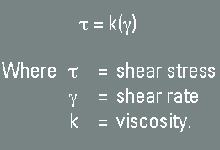# Newtonian fluid

1. . []

A typical fluid. A fluid is said to be Newtonian if its viscosity—a measure of a fluid’s ability to resist flow—only varies in response to changes in temperature or pressure. A Newtonian fluid will take the shape of its container.

Under constant temperature and pressure conditions, the viscosity of a Newtonian fluid is the constant of proportionality, or the ratio, between the shear stress that builds in the fluid to resist flow and the shear rate applied to the fluid to induce flow; the viscosity is the same for all shear rates applied to the fluid.

Water, sugar solutions, glycerin, silicone oils, light-hydrocarbon oils, air and other gases are Newtonian fluids. Most drilling fluids are non-Newtonian fluids.Share